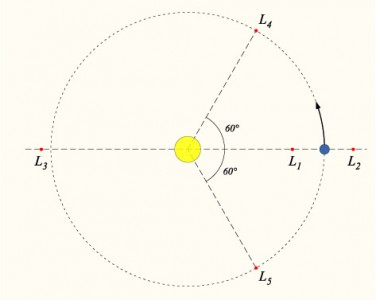# What is Lagrangian Point?

## Lagrangian Point

In Lagrangian mechanics, a Lagrangian point (or L-point) is one of five positions in space where the gravitational fields of two bodies of substantial but differing mass combine to form a point at which a third body of negligible mass would be stationary relative to the two bodies.

Bodies at the L-point will not move relative to the parent bodies if they are not perturbed by other gravitational forces. They are sometimes also referred to as libration points.

## The five points are labelled and defined as follows:

### L1

On the line defined by the two large masses, and between them.
Example: An object which orbits the Sun more closely than the Earth does would normally have a shorter orbital period than the Earth, but that ignores the effect of the Earth’s own gravitational pull. If the object is directly between the Earth and the Sun, then the effect of the Earth’s gravity is to weaken the force pulling the object towards the Sun, and therefore increase the orbital period of the object. The closer to Earth the object is, the greater this effect is. At a certain point, called the L1 point, the orbital period of the object becomes exactly equal to the Earth’s orbital period. The Solar and Heliospheric Observatory (SOHO) ( http://sohowww.nascom.nasa.gov/ ), for example, is stationed in a halo orbit around the Sun-Earth L1 point.

### L2

On the line defined by the two large masses, and beyond the smaller of the two.
Example: A similar effect occurs on the other side of the Earth, further away from the Sun, where the orbital period of an object would normally be greater than that of the Earth. The extra pull of the Earth’s gravity decreases the orbital period of the object, and at the L2 point that orbital period becomes equal to the Earth’s.

### L3

On the line defined by the two large masses, and beyond the larger of the two.
Example: A third Lagrangian point, L3, exists on the opposite side of the Sun, a little further away from the Sun than the Earth is, where the combined pull of the Earth and Sun again causes the object to orbit with the same period as the Earth. When used with the Sun and the Earth as the two masses, the L3 point was a popular place to put an “Anti-Earth” in pulp science fiction and comic books.

### L4

At the third point of an equilateral triangle with the base of the line defined by the two masses, such that the point is ahead of the smaller mass in its orbit around the larger mass.

### L5

At the third point of an equilateral triangle with the base of the line defined by the two masses, such that the point is behind the smaller mass in its orbit around the larger mass.
Example: The L4 and L5 points lie 60 degrees ahead of and 60 degrees behind the Earth in its orbit around the Sun. Unlike the other Lagrangian points, these points are resistant to perturbation, and therefore objects tend to accumulate around these points.

The latter two types of Lagrange points are sometimes called triangular Lagrange points or Trojan points.
In practice the stability of Lagrange points is not real, as there are more than three bodies in the universe. Additional gravitational pulls from elsewhere cause objects to move away from the point. The first three Langrangian points are stable only in the plane perpendicular to the line between the two bodies. This can be seen most easily by considering the L1 point.

## –

The Earth’s companion object 3753 Cruithne is in a somewhat Trojan-like orbit around the Earth, but not in the same manner as a true Trojan. It has a regular solar orbit that is bumped at times by Earth. When the asteroid approaches Earth, the asteroid takes orbital energy from Earth and moves into a larger, higher energy orbit.
When the asteroid (in a larger and slower orbit) is caught up by Earth, Earth takes the energy back and so the asteroid falls into a smaller, faster orbit and eventually catches Earth to begin the cycle anew. Epimetheus and Janus, satellites of Saturn, have a similar relationship, though they are of similar masses and so actually exchange orbits periodically. Another similar configuration is known as orbital resonance, in which orbiting bodies tend to have periods of a simple integer ratio, due to their interaction.

The Saturnian moon Tethys has two smaller moons in its L4 and L5 points, Telesto and Calypso. The Saturnian moon Dione has the moon Helene in its L4 point.
—–